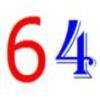# Resources tagged with: Calculators

Filter by: Content type:
Age range:
Challenge level:

There are 24 NRICH Mathematical resources connected to Calculators, you may find related items under Physical and Digital Manipulatives.

Broad Topics > Physical and Digital Manipulatives > Calculators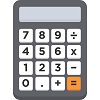### Secret Number

##### Age 5 to 7Challenge Level

Annie and Ben are playing a game with a calculator. What was Annie's secret number?##### Age 14 to 16Challenge Level

If a sum invested gains 10% each year how long before it has doubled its value?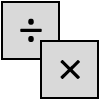### Four Go

##### Age 7 to 11Challenge Level

This challenge is a game for two players. Choose two of the numbers to multiply or divide, then mark your answer on the number line. Can you get four in a row?### Round and Round and Round

##### Age 11 to 14Challenge Level

Where will the point stop after it has turned through 30 000 degrees? I took out my calculator and typed 30 000 ÷ 360. How did this help?### Largest Product

##### Age 11 to 14Challenge Level

Which set of numbers that add to 10 have the largest product?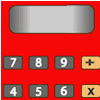### Forgot the Numbers

##### Age 7 to 11Challenge Level

On my calculator I divided one whole number by another whole number and got the answer 3.125. If the numbers are both under 50, what are they?### Climbing Powers

##### Age 16 to 18Challenge Level

$2\wedge 3\wedge 4$ could be $(2^3)^4$ or $2^{(3^4)}$. Does it make any difference? For both definitions, which is bigger: $r\wedge r\wedge r\wedge r\dots$ where the powers of $r$ go on for ever, or. . . .### Rain or Shine

##### Age 16 to 18Challenge Level

Predict future weather using the probability that tomorrow is wet given today is wet and the probability that tomorrow is wet given that today is dry.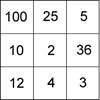### Four Go for Two

##### Age 7 to 11Challenge Level

Four Go game for an adult and child. Will you be the first to have four numbers in a row on the number line?### Number Pattern Explorations

##### Age 7 to 14Challenge Level

Investigate what happens if we create number patterns using some simple rules.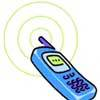### Mobile Numbers

##### Age 5 to 11Challenge Level

In this investigation, you are challenged to make mobile phone numbers which are easy to remember. What happens if you make a sequence adding 2 each time?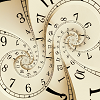### Decimal Time

##### Age 11 to 14Challenge Level

Use the clocks to investigate French decimal time in this problem. Can you see how this time system worked?### One Million to Seven

##### Age 7 to 11Challenge Level

Start by putting one million (1 000 000) into the display of your calculator. Can you reduce this to 7 using just the 7 key and add, subtract, multiply, divide and equals as many times as you like?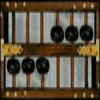##### Age 5 to 16

Nowadays the calculator is very familiar to many of us. What did people do to save time working out more difficult problems before the calculator existed?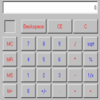### Calculating the Difference: A Discussion of the Use of Calculators in the English Primary Classroom.

##### Age 5 to 11

Clare Green looks at the role of the calculator in the teaching and learning of primary mathematics.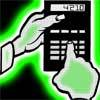### Reverse Trick

##### Age 7 to 11Challenge Level

Tell your friends that you have a strange calculator that turns numbers backwards. What secret number do you have to enter to make 141 414 turn around?### A Conversation Piece

##### Age 7 to 11Challenge Level

Take the number 6 469 693 230 and divide it by the first ten prime numbers and you'll find the most beautiful, most magic of all numbers. What is it?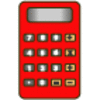### Clever Keys

##### Age 7 to 11 ShortChallenge Level

On a calculator, make 15 by using only the 2 key and any of the four operations keys. How many ways can you find to do it?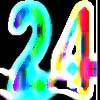### Special 24

##### Age 7 to 11Challenge Level

Find another number that is one short of a square number and when you double it and add 1, the result is also a square number.### Book Codes

##### Age 7 to 11Challenge Level

Look on the back of any modern book and you will find an ISBN code. Take this code and calculate this sum in the way shown. Can you see what the answers always have in common?### Triangle Incircle Iteration

##### Age 14 to 16Challenge Level

Keep constructing triangles in the incircle of the previous triangle. What happens?##### Age 11 to 14Challenge Level

Make a set of numbers that use all the digits from 1 to 9, once and once only. Add them up. The result is divisible by 9. Add each of the digits in the new number. What is their sum? Now try some. . . .